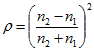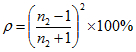Fresnel's formula

Fresnel's formula

Formula used to determine the amount of light reflected at the interface between two transparent media. The reflection iswhere n1 is the index of refraction of the first medium and n2 that of the second medium. For a lens surface in air, the percentage of light reflected is given byExample: the reflection from the two surfaces of a glass lens made of crown (n = 1.523) is equal to 8.6%. See ghost image; surface reflection.
 Table F3 Percentage of light reflected in normal incidence r at the surface of several transparent substances of varying refractive indices, in air refractive index ρ (%) 1.333 2.04 1.4 2.78 1.45 3.35 1.5 4.0 1.523 4.3 1.55 4.65 1.6 5.32 1.65 6.02 1.7 6.72 1.75 7.44 1.8 8.16
Millodot: Dictionary of Optometry and Visual Science, 7th edition. © 2009 Butterworth-Heinemann
Site: Follow: Share:
Open / Close